﻿ 二维方腔内HITEC熔盐熔化非稳态传热数值模拟 Unsteady Heat Transfer of HITEC Molten Salt in Two-Dimensional Square Cavity

Sustainable Energy
Vol.07 No.06(2017), Article ID:23003,11 pages
10.12677/SE.2017.76011

Unsteady Heat Transfer of HITEC Molten Salt in Two-Dimensional Square Cavity

Bo Wu1, Zhongxian Yuan1, Tianbao Sun2, Zhongqiu Liu1

1College of Environmental and Energy Engineering, Beijing University of Technology, Beijing

2National Institute of Metrology, Beijing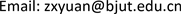Received: Nov. 22nd, 2017; accepted: Dec. 6th, 2017; published: Dec. 13th, 2017ABSTRACT

A numerical study has been conducted on the heat transfer of the molten HITEC salt, in which the natural convection heat transfer of the liquid salt was considered in the closed square cavity equipped with a heating bar. The two-dimensional heat transfer model of the molten salt was established and the problem was numerically solved with the FLUENT software. The dynamic change of the molten region as well as the melting fraction in the cavity was addressed in the condition of different heating power. The numerical result has revealed that it is considered mainly caused by the buoyancy-driven fluid flow of the molten salt and the vortex would enlarge with the molten range increasing. As the result of the coupled heat transfer of the natural convection and the conduction, the process of the salt melting can be divided into three stages, i.e. the heat conduction dominated stage at the beginning, the phase changing stage in the middle period, and the convection dominated stage in the last. The temperature distribution in the square cavity is obviously different in different stage. Either increasing the heating power or enlarge the heating bar size are all helpful to the salt melting process. Nevertheless, changing the heating bar size seems to be more effective for the melting dynamics in comparison.

Keywords:Molten Salt, Natural Convection, Phase Change, Heat Transfer, Numerical Simulation

1北京工业大学环境与能源工程学院，北京

2中国计量科学研究院，北京1. 引言

2. 物理模型

1) 熔盐不可压缩，各向同性，与外界环境不发生任何物质交换；

2) 在材料熔化区间，熔盐的物性参数采用温度的函数，其具体形式在下文叙述；

3) 液相的流动为层流；

4) 熔盐内部初始温度分布均匀。

3. 数值模型

FLUENT软件中凝固熔化模型(Solidification/Melting Model)是由Voller和Prakash等提出，它被称为“计算材料凝固熔化的焓–多孔法”   。在该方法中，熔融界面并不被跟踪，而是在数值分析相变时，计算区域采用材料的多孔性进行表征，其特征在于引入液相系数β，其定义为

$\beta =\left\{\begin{array}{ll}0\hfill & \text{\hspace{0.17em}}\text{\hspace{0.17em}}\text{\hspace{0.17em}}T<{T}_{s}\hfill \\ \frac{T-{T}_{s}}{{T}_{l}-{T}_{s}}\hfill & {T}_{s} (1)Figure 1. Geometric model of square cavity in the simulation

3.1. 数学描述

1) 连续性方程

$\frac{\partial \rho }{\partial t}+\nabla \cdot \left(\rho V\right)=0$ (2)

2) 动量方程

$\frac{\partial \left(\rho u\right)}{\partial t}+\nabla \cdot \left(\rho uV\right)=\nabla \cdot \left(\mu \nabla u\right)+{S}_{u}$ (3)

$\frac{\partial \left(\rho v\right)}{\partial t}+\nabla \cdot \left(\rho vV\right)=\nabla \cdot \left(\mu \nabla v\right)+{S}_{v}$ (4)

${S}_{u}=\frac{{\left(1-\beta \right)}^{2}}{{\beta }^{3}+\epsilon }{A}_{mush}u-\frac{\partial p}{\partial x}$ (5)

${S}_{v}=\frac{{\left(1-\beta \right)}^{2}}{{\beta }^{3}+\epsilon }{A}_{mush}v-\frac{\partial p}{\partial y}-{\rho }_{ref}g\left[1-\alpha \left(T-{T}_{ref}\right)\right]$ (6)

3) 能量方程

$\frac{\partial }{\partial t}\left(\rho H\right)+\nabla \cdot \left(\rho VH\right)=\nabla \cdot \left(k\nabla T\right)$ (7)

$H=h+\Delta H$ (8)

$h={h}_{ref}+{\int }_{{T}_{ref}}^{T}{C}_{p}\text{d}T$ (9)

$\Delta H=\beta L$ (10)

3.2. 初始条件与边界条件

$r=0.0\text{5m}$ 的圆表面上，

$-k\frac{\partial T}{\partial r}=q$ (11)

3.3. 熔盐HITEC的物性参数

$\rho =2287.7993-0.748T$ (12)

${C}_{p}=1507-0.1T$ (13)

$\lambda =0.8099-7.827×{10}^{-4}T+1.043×{10}^{-7}{T}^{2}$ (14)

$\eta =0.06248-2.253×{10}^{-4}T+2.7903×{10}^{-7}{T}^{2}-1.1738×{10}^{-10}{T}^{3}$ (15)

3.4. 网格划分

4. 计算结果分析

4.1. 相界面变化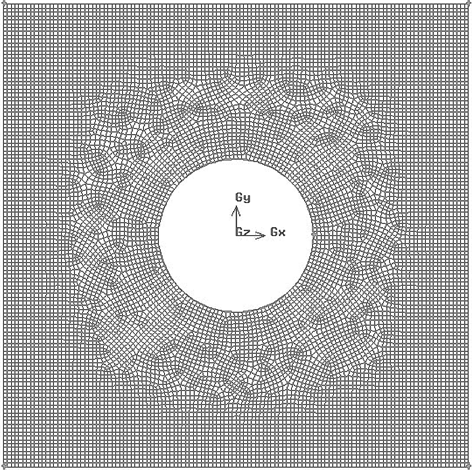Figure 2. Schematic of the computational grid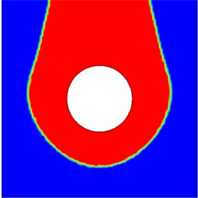(a) 2.8 h(b) 8.4 h(c) 11.2 h(d) 14.0 h(e) 19.6 h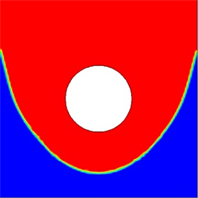(f) 22.4 h

Figure 3. The interface change over time when q = 3000 W/m2, Red presents liquid state, blue presents solid state

4.2. 不同时刻温度分布(a) 2.8 h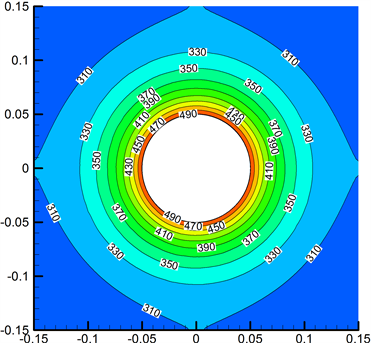(b) 8.4 h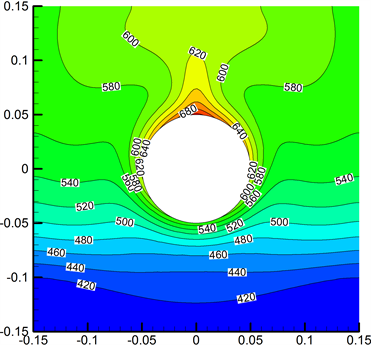(c) 11.2 h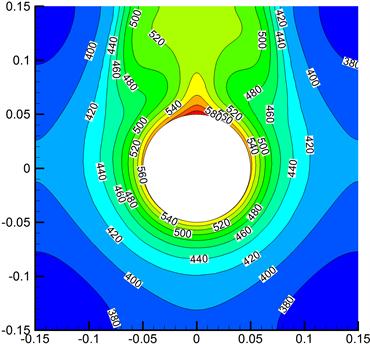(d) 19.6 h

Figure 4. Temperature distribution of the molten salt at different times

4.3. 不同时刻的流场

4.4. 熔盐液相比变化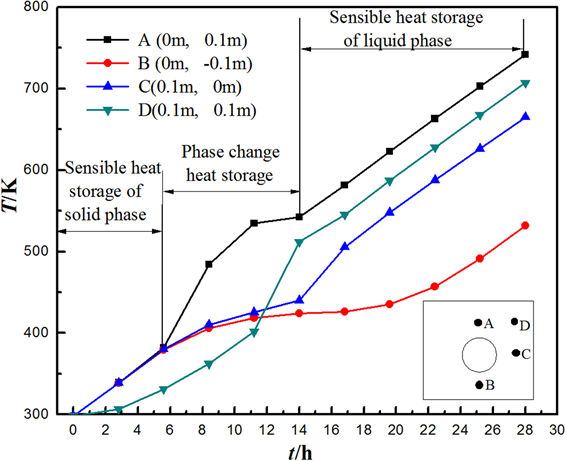Figure 5. Temperature variation of the monitored points during the melting process at q = 3000 W/m2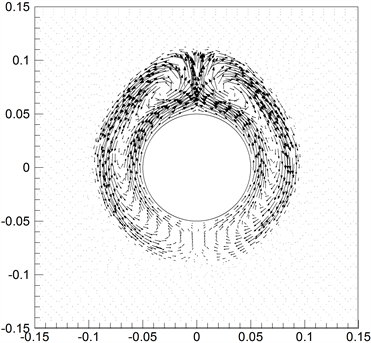(a) 2.8 h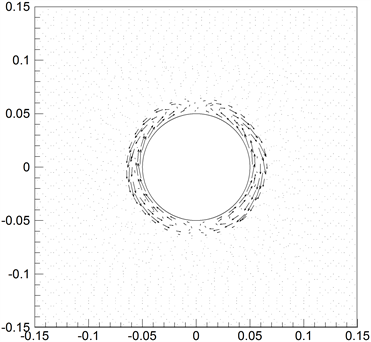(b) 8.4 h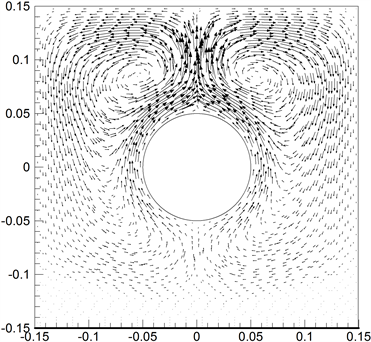(c) 11.2 h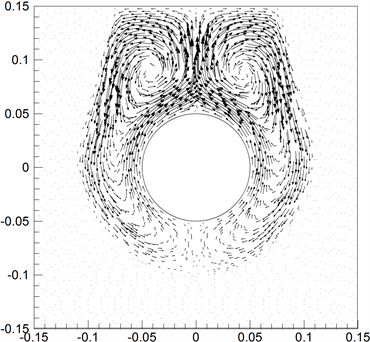(d) 19.6 h

Figure 6. The flow patterns of the molten salt at different heating time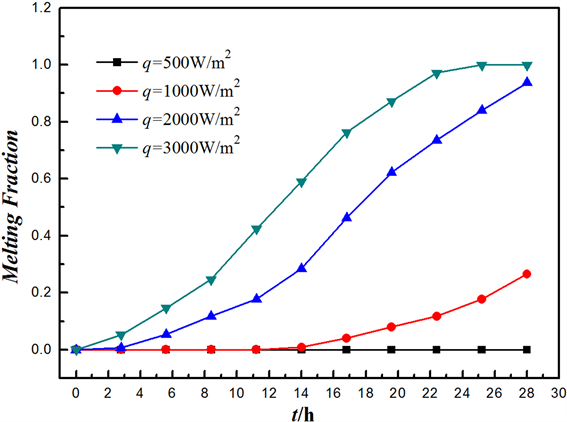Figure 7. The profile of the melting fraction for different heating power at d = 10 cm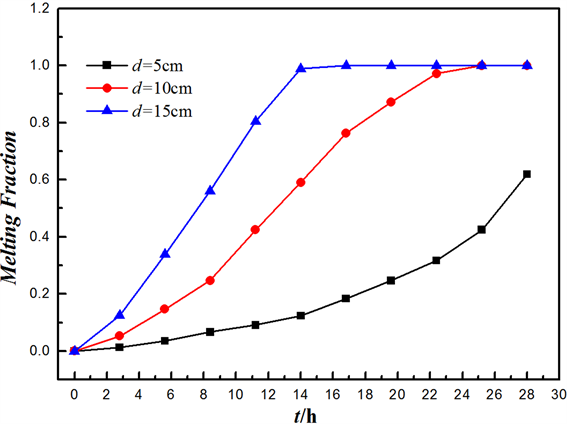Figure 8. The change of melting fraction for different heating bar size at q = 3000 W/m2

5. 结论

Unsteady Heat Transfer of HITEC Molten Salt in Two-Dimensional Square Cavity[J]. 可持续能源, 2017, 07(06): 97-107. http://dx.doi.org/10.12677/SE.2017.76011

1. 1. International Energy Agency (2016) International Energy Agency (IEA)’s World Energy Outlook.

2. 2. Agyenim, F., Hewitt, N., Eames, P., et al. (2010) A Review of Materials, Heat Transfer and Phase Change Problem Formulation for Latent Heat Thermal Energy Storage Systems (LHTESS). Renewable and Sustainable Energy Reviews, 14, 615-628.

3. 3. 夏红德, 张华良, 徐玉杰, 等. 我国工业节能潜力与对策分析[J]. 工程热物理学报, 2011, 32(12): 1992-1996.

4. 4. Xu, B., Li, P. and Chan, C. (2015) Application of Phase Change Materials for Thermal Energy Storage in Concentrated Solar Thermal Power Plants: A Review to Recent Developments. Applied Energy, 160, 286-307.

5. 5. Vyshak, N.R. and Jilani, G. (2007) Numerical Analysis of Latent Thermal Energy Storage System. Energy Conversion and Management, 48, 2161-2168.

6. 6. 菅鲁京, 张加迅, 李劲东. 自然对流对相变材料熔化过程的影响分析[J]. 中国空间科学技术, 2009(2): 59-64.

7. 7. Hussain, S.H. and Hussein, A.K. (2010) Numerical Investigation of Natural Convection Phenomena in a Uniformly Heated Circular Cylinder Immersed in Square Enclosure Filled with Air at Different Vertical Locations. International Communications in Heat and Mass Transfer, 37, 1115-1126.

8. 8. Sheremet, M.A., Pop, I. and Rahman, M.M. (2015) Three-Dimensional Natural Convection in a Porous Enclosure Filled with a Nano-Fluid Using Buongiorno’s Mathematical Model. International Journal of Heat and Mass Transfer, 82, 396-405.

9. 9. Casella, E. and Giangi, M. (2001) An Analytical and Numerical Study of the Stefan Problem with Convection by Means of an Enthalpy Method. Mathematical Methods in the Applied Sciences, 24, 623-639.

10. 10. 杨卫卫, 何雅玲, 徐超, 等. 二维方腔非稳态自然对流数值模拟研究[J]. 工程热物理学报, 2004, 25(2): 281-283.

11. 11. Dong, S.F. and Li, Y.T. (2004) Conjugate of Natural Convection and Conduction in a Complicated Enclosure. International Journal of Heat and Mass Transfer, 47, 2233-2239.

12. 12. 张敏, 晏刚, 陶锴. 内置发热体的封闭方腔自然对流换热数值模拟[J]. 化工学报, 2010, 61(6): 1373-1378.

13. 13. 李世武, 熊莉芳. 封闭方腔自然对流换热研究[J]. 工业加热, 2007, 36(3): 10-13.

14. 14. Crank, J. (1984) Free and Moving Boundary Problems. Clarendon Press, Oxford.

15. 15. Voller, V.R. and Prakash, C. (1987) A Fixed-Grid Numerical Modeling Methodology for Convection-Diffusion Mushy Region Phase Change Problems. International Journal Heat and Mass Transfer, 30, 1709-1719. https://doi.org/10.1016/0017-9310(87)90317-6

16. 16. Voller, V.R. and Swaminathan, C.R. (1991) Generalized Source-Based Method for Solidification Phase Change. Numerical Heat Transfer Part B, 19, 175-189. https://doi.org/10.1080/10407799108944962

17. 17. Chen, X., Wang, C., Wu, Y.T., et al. (2016) Numerical Simulation of Mixed Convection Heat Transfer of Molten Salt in Horizontal Square Tube with Single Surface Heating. Applied Thermal Engineering, 104, 282-293.